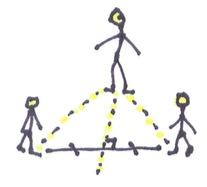Kinesthetics

# Kinesthetic DistanceHenri Picciotto

(Disclaimer: I have done most of these activities with students, but not all of them.)

These activities are intended for a geometry class. They are best done in a gym or playground, before discussing circles, perpendicular bisectors, angle bisectors, etc. It may be best to not do all of the activities in one session.

1. Choose a student to be Point A. Ask the others to stand so that they are all at the same distance from Point A. Hopefully, they'll make a circle. Introduce the term "locus": the locus of points at a given distance from A is a circle with A as its center. (I usually say that "locus" is the mathematical term for "location". You can also just say "location" and wait for a future class to introduce "locus".)
2. Choose two students to be points A and B. Ask the others to stand so that they are
• closer to A than to B
• closer to B than to A
• equidistant from A and B
(Explain the word "equidistant".) Discuss the properties of the locus (it is the perpendicular bisector.)
3. Choose three students to be points A, B, and C. Ask the others to stand so that they are
• closer to A than to B or C
• closer to B than to A or C
• closer to C than to A or B
• equidistant from A, B, and C
The latter will not be possible for a large group. Ask everyone but A, B, and C to stand aside, and ask for a volunteer to stand equidistant from the three. Ask others to give advice. If this works, name this student Point O, and ask additional students to stand at the same distance from O as A, B, and C are. Discuss the locus (the circumscribed circle.)
4. Use a line on the floor or on the ground. Ask students to stand so that they are all at the same distance from the line. Discuss what "distance to a line" might mean. (If necessary, point out that a point's distance to a point on the line depends on which point is chosen on the line. What choice would make sense?)
5. Choose one of the students to be Point O, not on the line. Have another student stand on the line, as close to O as possible. Name that student Point P. Ask others to stand at the same distance from O as P is. Discuss the locus (a circle centered at O, tangent to the line.)
6. Use two intersecting lines on the floor or ground. If in a gym, you'll probably have to use existing painted perpendicular lines. If on the playground, you might be able to draw your own lines using carpenter's chalk. Name the lines L1 and L2. Ask students to stand:
• closer to L1 than to L2
• closer to L2 than to L1
• equidistant from L1 and L2
Discuss the properties of the locus (the angle bisector.)

### Extension beyond the usual geometry topics:

1. Ask a student to be point F. Have that student stand a few steps away from a line. Ask students to stand
• closer to the line than to F
• closer to F than to the line
• equidistant from F and the line
This is quite a bit more difficult than the previous exercises. You may need to have a few students take the role of distance judges: counting steps from a given student to the line and to F and helping him/her move if necessary. Once the locus (a parabola) has been found, discuss.
2. For this one, you will need a very long string. Ask two students to be points F1 and F2. They will each hold one end of the string close to their body. Ask other students to use the string in turn to find spots so that the sum of the distances to F1 and F2 is equal to the length of the string. The locus is an ellipse. Discuss.